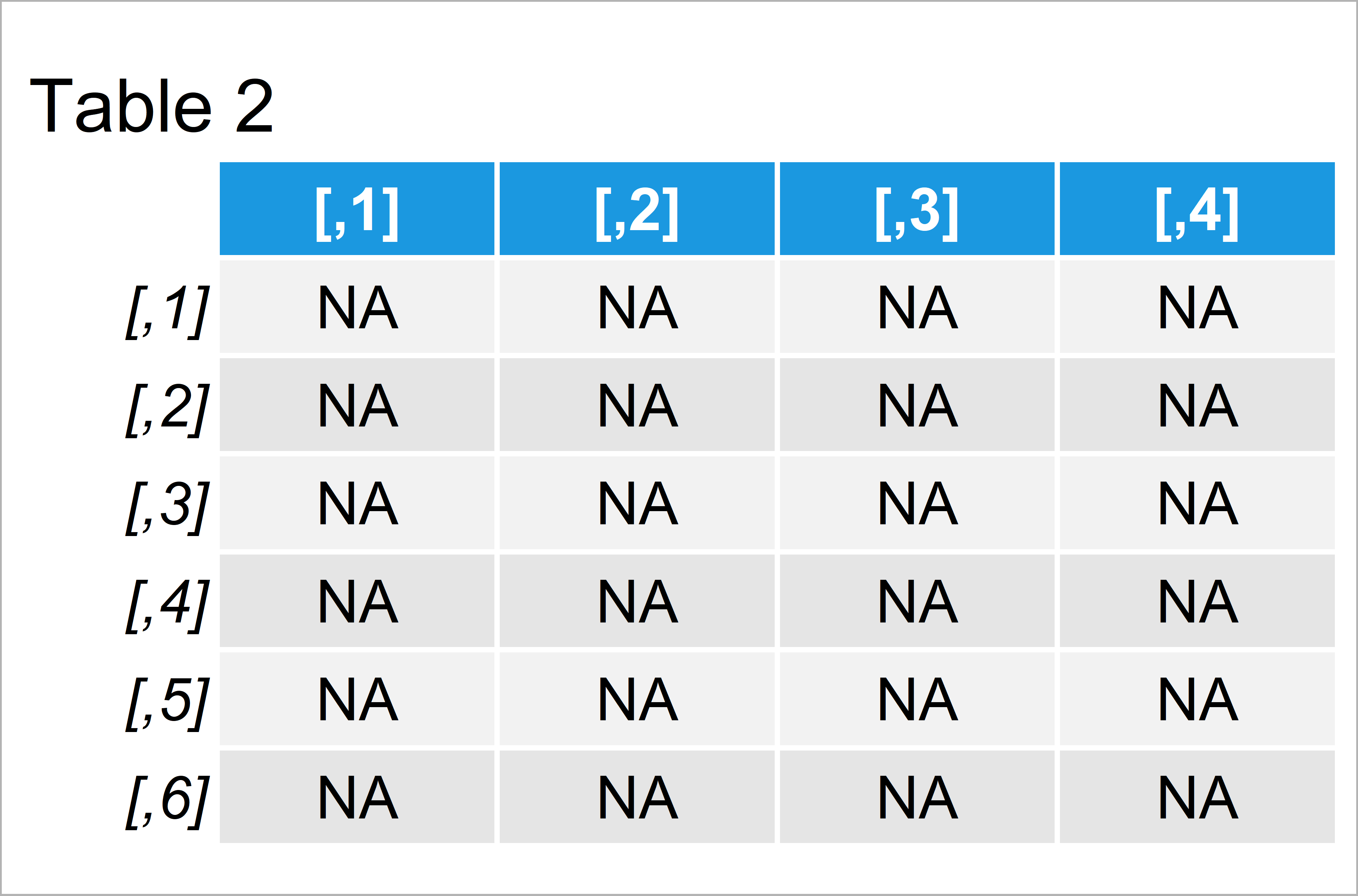# Create Matrix that Only Contains Zeros in R (2 Examples)

In this R tutorial you’ll learn how to construct a matrix that consists only of zeros (i.e. 0).

Let’s take a look at some R codes in action.

## Example 1: Create Matrix Containing Only Zeros

The following R programming syntax shows how to fill a matrix with zeros only.

For this, we can apply the matrix function as well as the ncol and nrow arguments.

Within the matrix function, we only have to specify the value 0 once. This value is automatically replicated to fill our matrix according to the ncol and nrow arguments.

Have a look at the R code below:

```mat_zero <- matrix(0, ncol = 4, nrow = 6)    # Create zero-matrix
mat_zero                                     # Print zero-matrix```The output of the previous R programming code is shown in Table 1: A matrix containing only the value 0.

This is basically all you need to know to create a zero-matrix in R. However, I just want to explain the logic of the previous R code in some more detail, so keep on reading!

## Example 2: Create Matrix Containing Only NA Values

The syntax below explains how to fill up a matrix with NA values instead of zeros.

For this, we simply have to replace 0 in the code that I have explained in Example 1 with an NA:

```mat_NA <- matrix(NA, ncol = 4, nrow = 6)     # Create NA-matrix
mat_NA                                       # Print NA-matrix```Table 2 shows the output of the previous R code – An empty matrix containing only missing values.

Think about the logic of this: You can basically insert any value at the beginning of the matrix function and is duplicated as often as necessary to fill in all the data cells that we are specifying with the ncol and nrow functions.

You could also use this code to create a matrix of ones, twos, letters, names, or whatever you want.

## Video & Further Resources

In case you need more explanations on the R programming syntax of this page, you might watch the following video of my YouTube channel. I illustrate the examples of this tutorial in the video.

Please accept YouTube cookies to play this video. By accepting you will be accessing content from YouTube, a service provided by an external third party.If you accept this notice, your choice will be saved and the page will refresh.

In addition, you may want to have a look at the related articles of this website.

To summarize: In this R tutorial you have learned how to create a zero-matrix. Let me know in the comments section, if you have further questions.

Subscribe to the Statistics Globe Newsletter